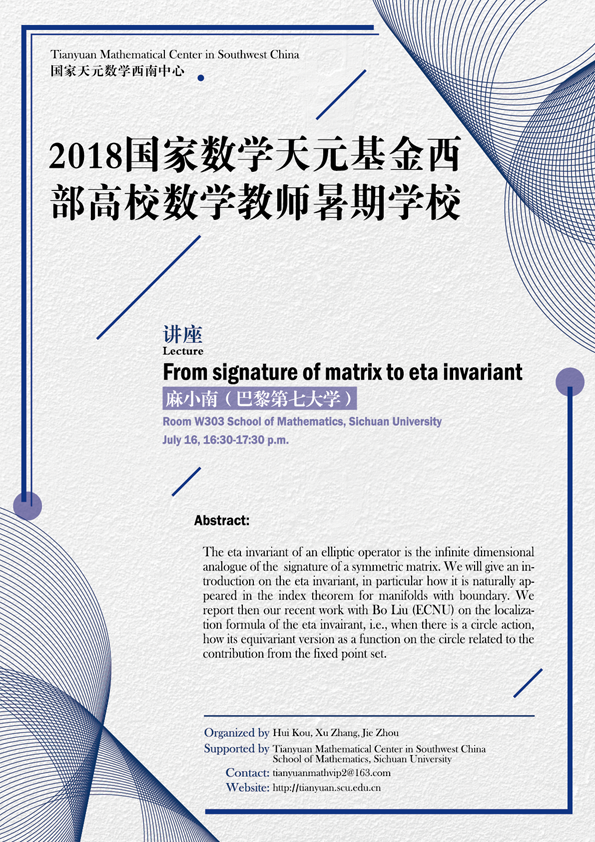From signature of matrix to eta invariant

#### W303  School of Mathematics, Sichuan University#### ABSTRACT

The eta invariant of an elliptic operator is the infinite dimensional analogue of the signature of a symmetric matrix. We will give an introduction on the eta invariant, in particular how it is naturally appeared in the index theorem for manifolds with boundary. We report then our recent work with Bo Liu (ECNU) on the localization formula of the eta invairant, i.e., when there is a circle action, how its equivariant version as a function on the circle related to the contribution from the fixed point set.

#### ORGANIZERS

Hui Kou (Sichuan University)

Xu Zhang (Sichuan University)

Jie Zhou (Sichuan University)

#### SUPPORTED BY

Tianyuan Mathematical Center in Southwest China

School of Mathematics, Sichuan University

#### VIDEO

• From signature of matrix to eta invariant
• 16:30 - 17:30, 2018-07-16
• 麻小南（巴黎第七大学）